# FED DERIVATIVES TAPERING CURRENCY Understanding Currency Derivatives By

• Slides: 18FED DERIVATIVES TAPERING CURRENCY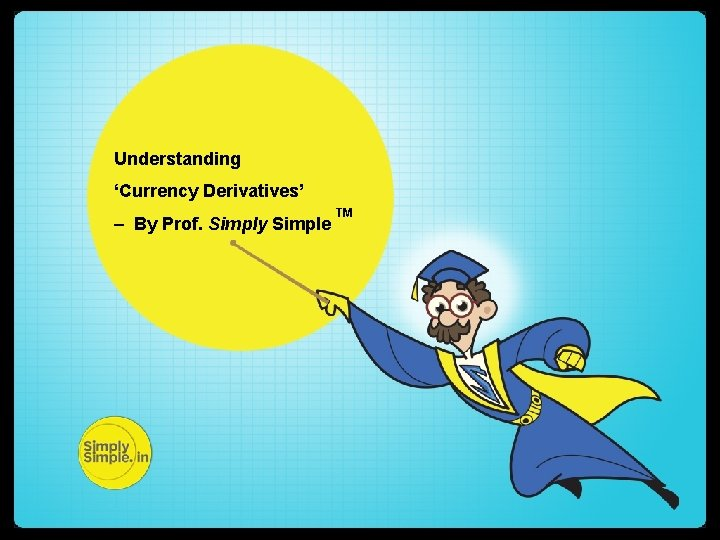Understanding ‘Currency Derivatives’ – By Prof. Simply Simple TM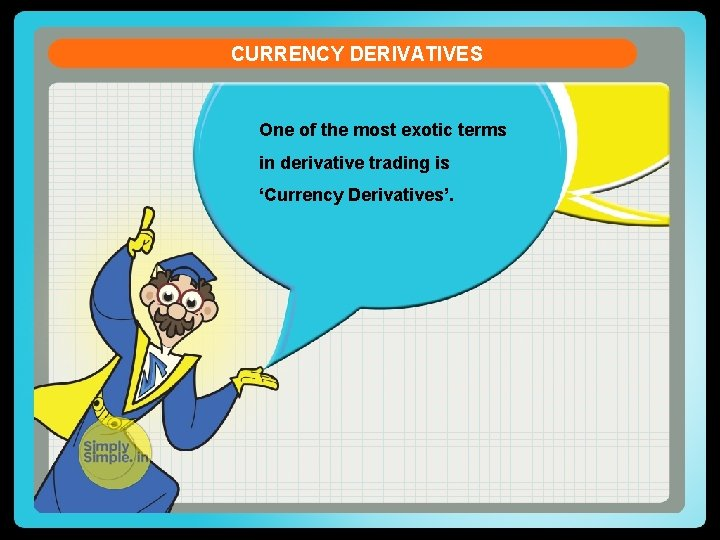CURRENCY DERIVATIVES One of the most exotic terms in derivative trading is ‘Currency Derivatives’.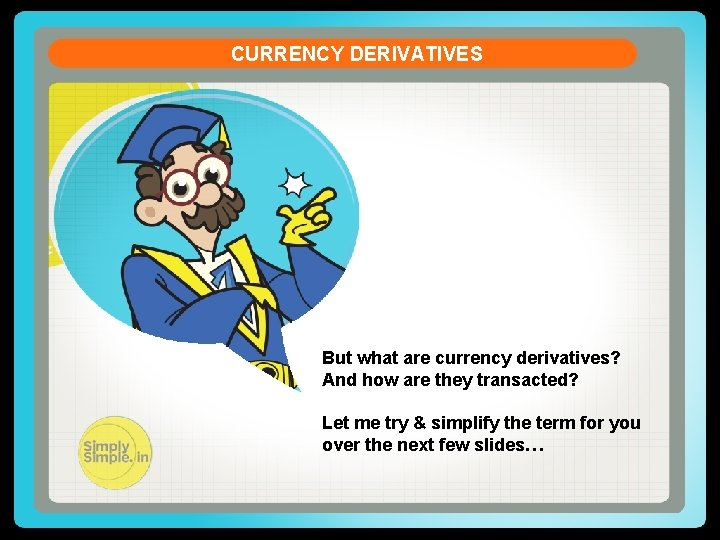CURRENCY DERIVATIVES But what are currency derivatives? And how are they transacted? Let me try & simplify the term for you over the next few slides…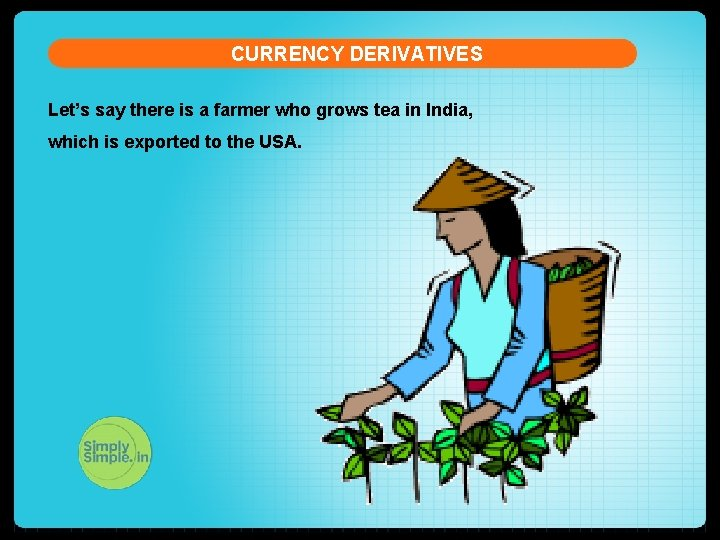CURRENCY DERIVATIVES Let’s say there is a farmer who grows tea in India, which is exported to the USA.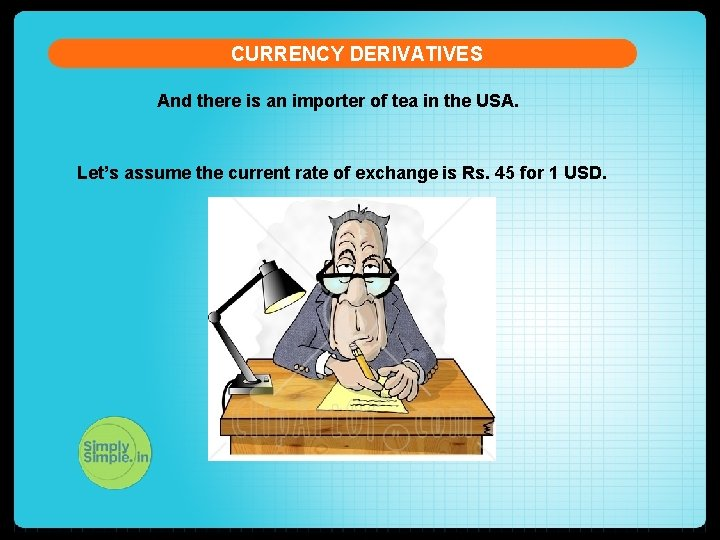CURRENCY DERIVATIVES And there is an importer of tea in the USA. Let’s assume the current rate of exchange is Rs. 45 for 1 USD.CURRENCY DERIVATIVES Assume that the tea grower agrees to supply 10 quintals of tea to the importer at 10 dollars a quintal three months down the line upon harvesting. (1 Quintal = 100 kgs) It is important to understand that the importer buys tea at 10 dollars a quintal, no matter what the exchange rate is.CURRENCY DERIVATIVES q The tea grower thinks that the rate of exchange, which is currently trading at Rs. 45 to a US dollar, could fall to Rs. 44 in 3 months. q This means that while the importer would pay her 100 dollars ( \$10 per quintal x 10 quintals = \$ 100), she would earn only Rs. 4400 ( \$100 x Rs 44 per dollar) instead of Rs 4500 ( \$100 x Rs 45 per dollar) thus incurring a loss of Rs. 100. ( Rs 4500 –Rs 4400)CURRENCY DERIVATIVES q So the tea grower goes to a currency trader and signs a ‘forward contract’ which says that at the end of 3 months the currency trader would hedge her against a possible decrease in exchange rates. q This means that, at the end of 3 months, the currency trader would pay her Rs. 4500 for her 100 USD, no matter what the prevailing exchange rate.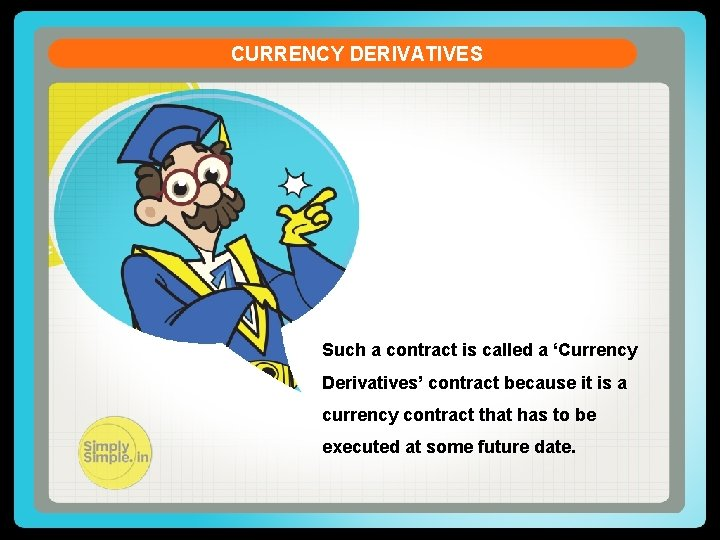CURRENCY DERIVATIVES Such a contract is called a ‘Currency Derivatives’ contract because it is a currency contract that has to be executed at some future date.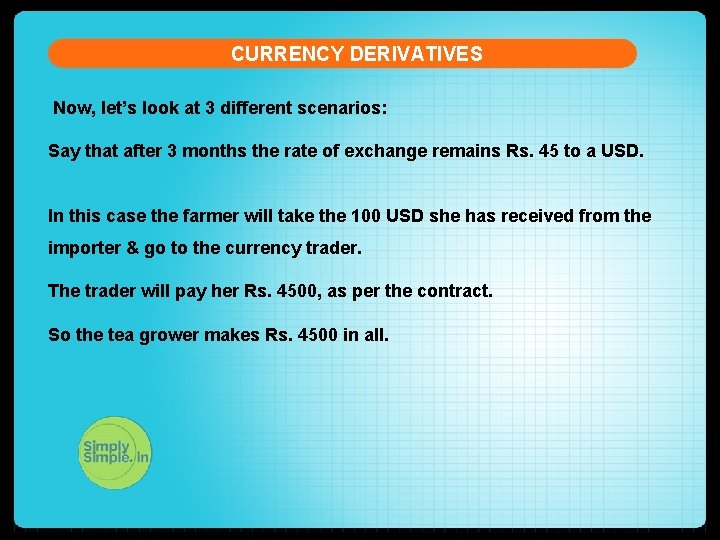CURRENCY DERIVATIVES Now, let’s look at 3 different scenarios: Say that after 3 months the rate of exchange remains Rs. 45 to a USD. In this case the farmer will take the 100 USD she has received from the importer & go to the currency trader. The trader will pay her Rs. 4500, as per the contract. So the tea grower makes Rs. 4500 in all.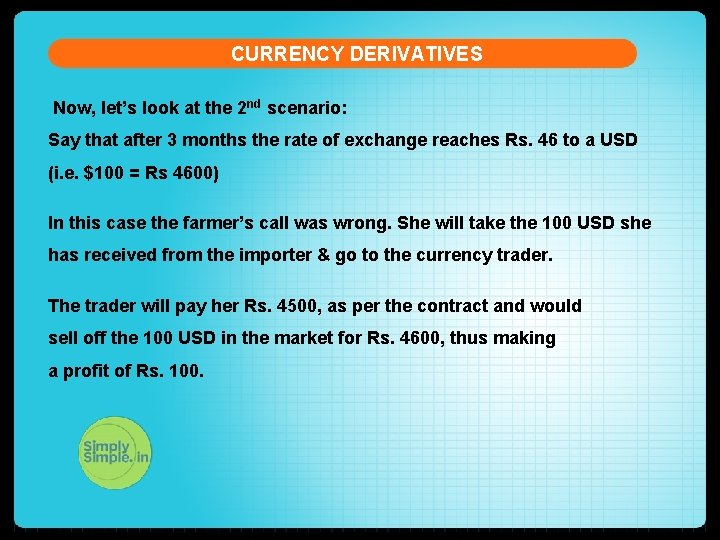CURRENCY DERIVATIVES Now, let’s look at the 2 nd scenario: Say that after 3 months the rate of exchange reaches Rs. 46 to a USD (i. e. \$100 = Rs 4600) In this case the farmer’s call was wrong. She will take the 100 USD she has received from the importer & go to the currency trader. The trader will pay her Rs. 4500, as per the contract and would sell off the 100 USD in the market for Rs. 4600, thus making a profit of Rs. 100.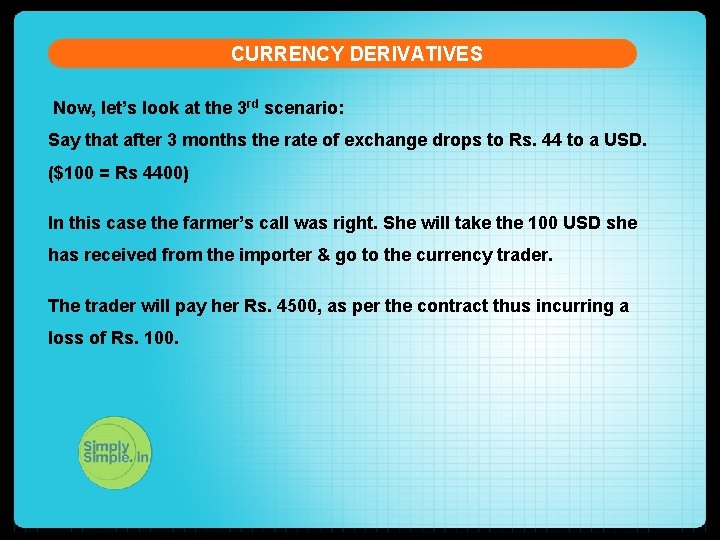CURRENCY DERIVATIVES Now, let’s look at the 3 rd scenario: Say that after 3 months the rate of exchange drops to Rs. 44 to a USD. (\$100 = Rs 4400) In this case the farmer’s call was right. She will take the 100 USD she has received from the importer & go to the currency trader. The trader will pay her Rs. 4500, as per the contract thus incurring a loss of Rs. 100.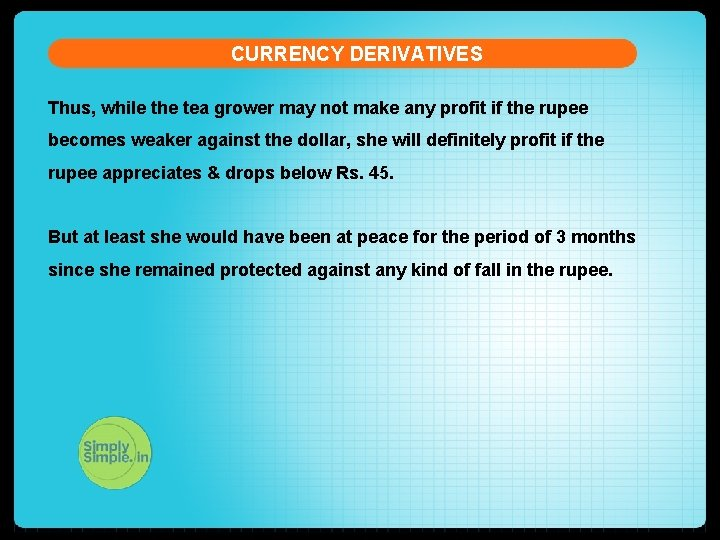CURRENCY DERIVATIVES Thus, while the tea grower may not make any profit if the rupee becomes weaker against the dollar, she will definitely profit if the rupee appreciates & drops below Rs. 45. But at least she would have been at peace for the period of 3 months since she remained protected against any kind of fall in the rupee.CURRENCY DERIVATIVES Hope you have now clearly understood the meaning of ‘currency derivatives’ and its practical application.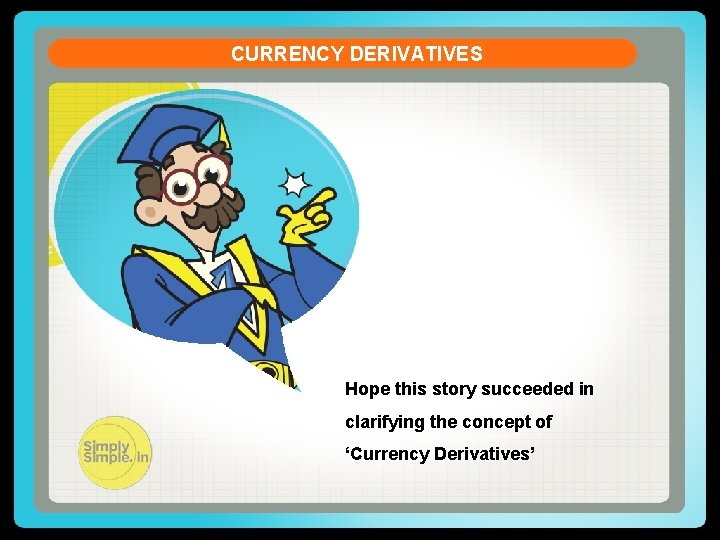CURRENCY DERIVATIVES Hope this story succeeded in clarifying the concept of ‘Currency Derivatives’